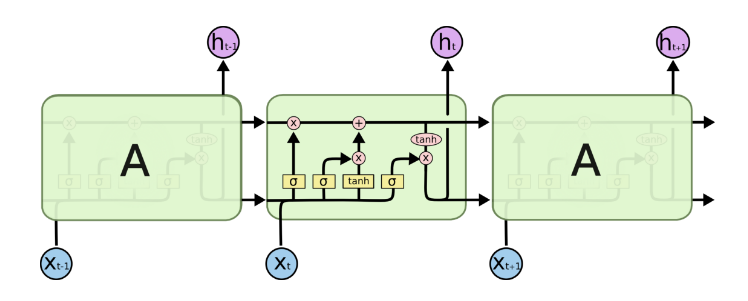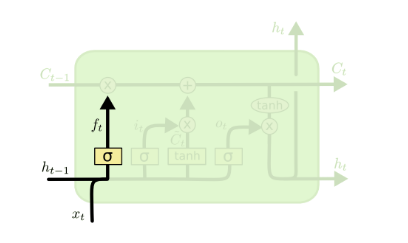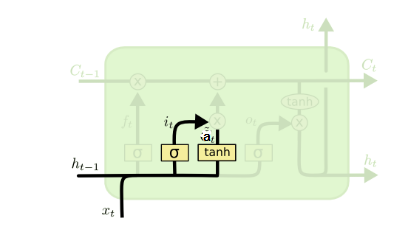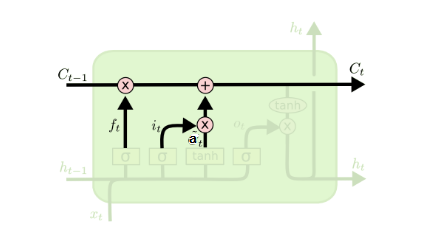# 1. 从RNN到LSTM# 2. LSTM模型结构剖析## 2.1 LSTM之遗忘门## 2.2 LSTM之输入门$i^{(t)} = \sigma(W_ih^{(t-1)} + U_ix^{(t)} + b_i)$

$a^{(t)} =tanh(W_ah^{(t-1)} + U_ax^{(t)} + b_a)$

## 2.3 LSTM之细胞状态更新## 2.4 LSTM之输出门# 3. LSTM前向传播算法

1）更新遗忘门输出：$f^{(t)} = \sigma(W_fh^{(t-1)} +U_fx^{(t)} + b_f)$

2）更新输入门两部分输出：$i^{(t)} = \sigma(W_ih^{(t-1)} + U_ix^{(t)} + b_i)a^{(t)} = tanh(W_ah^{(t-1)} + U_ax^{(t)} + b_a)$

3）更新细胞状态：$C^{(t)} = C^{(t-1)} \odot f^{(t)} + i^{(t)} \odot a^{(t)}$

4）更新输出门输出：$o^{(t)} = \sigma(W_oh^{(t-1)} + U_ox^{(t)} + b_o)h^{(t)} = o^{(t)} \odot tanh(C^{(t)})$

5）更新当前序列索引预测输出：$\hat{y}^{(t)} = \sigma(Vh^{(t)} + c)$

# 4. LSTM反向传播算法推导关键点

$\delta_h^{(t)} = \frac{\partial L}{\partial h^{(t)}}$

$\delta_C^{(t)} = \frac{\partial L}{\partial C^{(t)}}$

$\delta_h^{(\tau)} =\frac{\partial L}{\partial o^{(\tau)}} \frac{\partial o^{(\tau)}}{\partial h^{(\tau)}} = V^T(\hat{y}^{(\tau)} - y^{(\tau)})$

$\delta_C^{(\tau)} =\frac{\partial L}{\partial h^{(\tau)}} \frac{\partial h^{(\tau)}}{\partial C^{(\tau)}} = \delta_h^{(\tau)} \odot o^{(\tau)} \odot (1 - tanh^2(C^{(\tau)}))$

$\delta_h^{(t)}$的反向推导和RNN中的类似，因为它的梯度误差由前一层$\delta_h^{(t+1)}$的梯度误差和本层的输出梯度误差两部分组成，即：$\delta_h^{(t)} =\frac{\partial L}{\partial o^{(t)}} \frac{\partial o^{(t)}}{\partial h^{(t)}} + \frac{\partial L}{\partial h^{(t+1)}}\frac{\partial h^{(t+1)}}{\partial h^{(t)}} = V^T(\hat{y}^{(t)} - y^{(t)}) + W^T\delta^{(t+1)}diag(1-(h^{(t+1)})^2)$

$\delta_C^{(t)}$的反向梯度误差由前一层$\delta_C^{(t+1)}$的梯度误差和本层的从$h^{(t)}$传回来的梯度误差两部分组成，即：$\delta_C^{(t)} =\frac{\partial L}{\partial C^{(t+1)}} \frac{\partial C^{(t+1)}}{\partial C^{(t)}} + \frac{\partial L}{\partial h^{(t)}}\frac{\partial h^{(t)}}{\partial C^{(t)}} = \delta_C^{(t+1)}\odot f^{(t+1)} + \delta_h^{(t)} \odot o^{(t)} \odot (1 - tanh^2(C^{(t)}))$

# 5. LSTM小结

LSTM虽然结构复杂，但是只要理顺了里面的各个部分和之间的关系，进而理解前向反向传播算法是不难的。当然实际应用中LSTM的难点不在前向反向传播算法，这些有算法库帮你搞定，模型结构和一大堆参数的调参才是让人头痛的问题。不过，理解LSTM模型结构仍然是高效使用的前提。